# Digital to Analog Encoding 1 2 Digital modulation

• Slides: 25Digital to Analog Encoding 1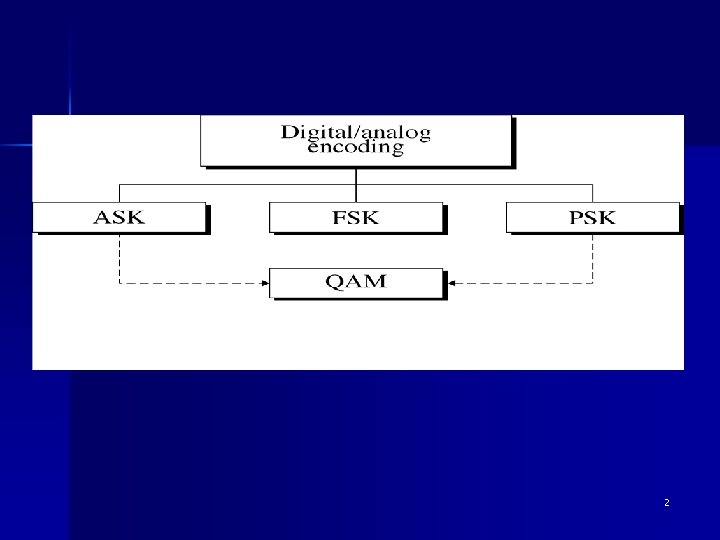2Digital modulation techniques Amplitude Shift Keying n Frequency Shift Keying n Phase Shift Keying n Quadrature Amplitude Modulation (QAM) n 3Amplitude Shift Keying n ASK – The strength of carrier signal varies (the amplitude changes) – The frequency and phase remain constant – The speed of transmission is limited by the physical characteristics of the transmission media – Susceptible to noise interference n (noise = unintentional voltages introduced onto a line by other sources, heat, etc. ) 4Amplitude Shift Keying (ASK) 5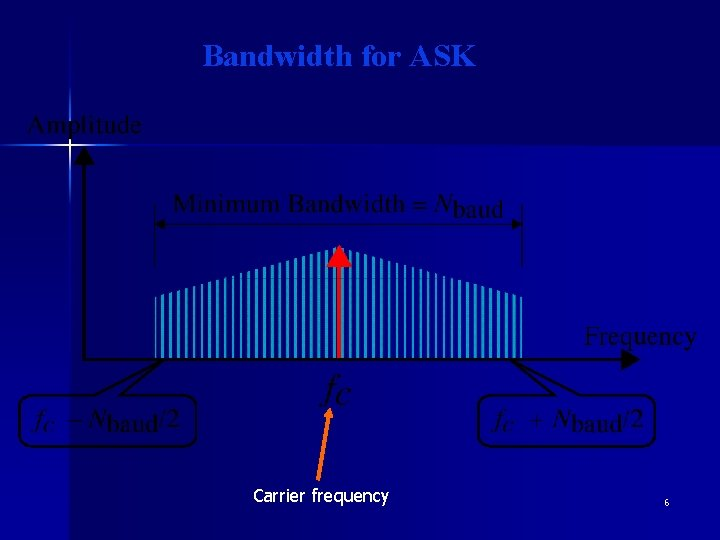Bandwidth for ASK Carrier frequency 6Frequency Shift Keying The frequency of the carrier varies n Amplitude and phase remain constant n 7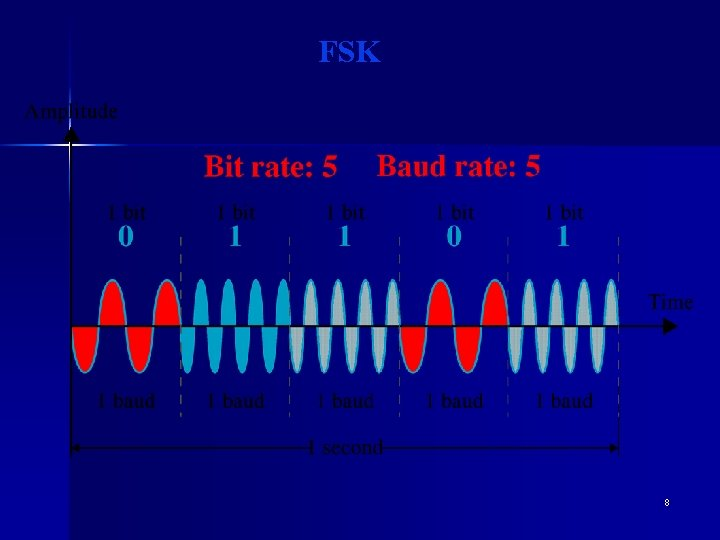FSK 8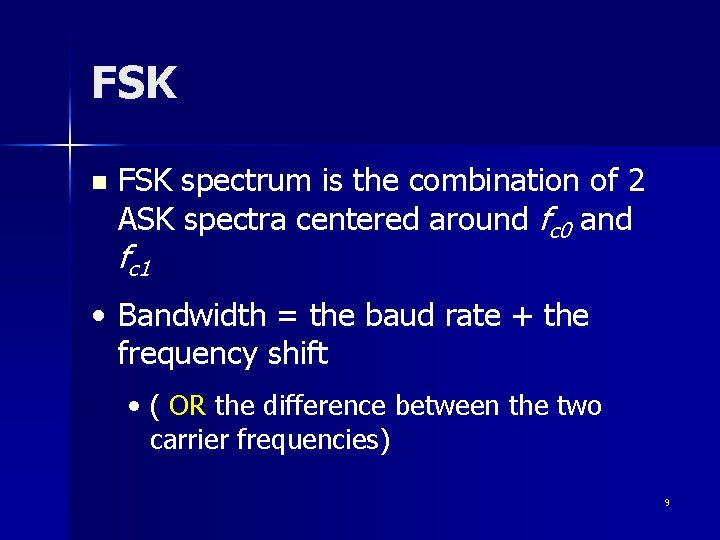FSK n FSK spectrum is the combination of 2 ASK spectra centered around fc 0 and fc 1 • Bandwidth = the baud rate + the frequency shift • ( OR the difference between the two carrier frequencies) 9Bandwidth for FSK Bandwidth = the baud rate + the frequency shift ( OR the difference between the two carrier frequencies) 10Phase Shift Keying The phase of the carrier varied n The amplitude and frequency remain constant n 11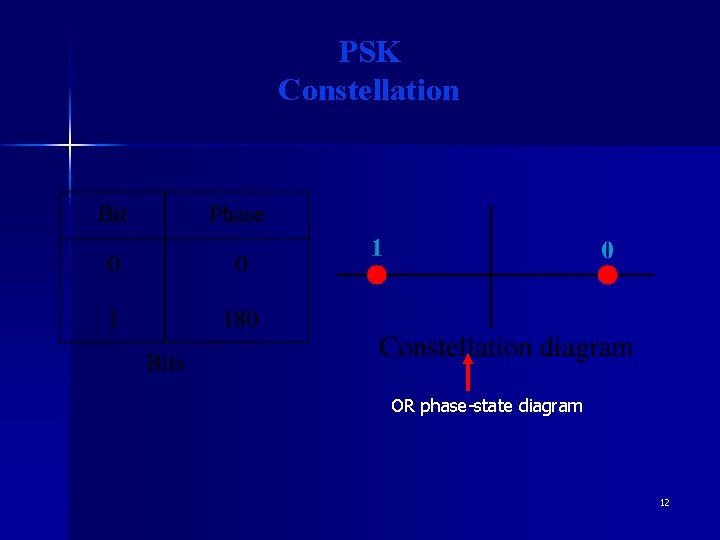PSK Constellation OR phase-state diagram 12PSK Example : if a phase 0 degree represents binary 0 change the phase to 180 degree binary 1 13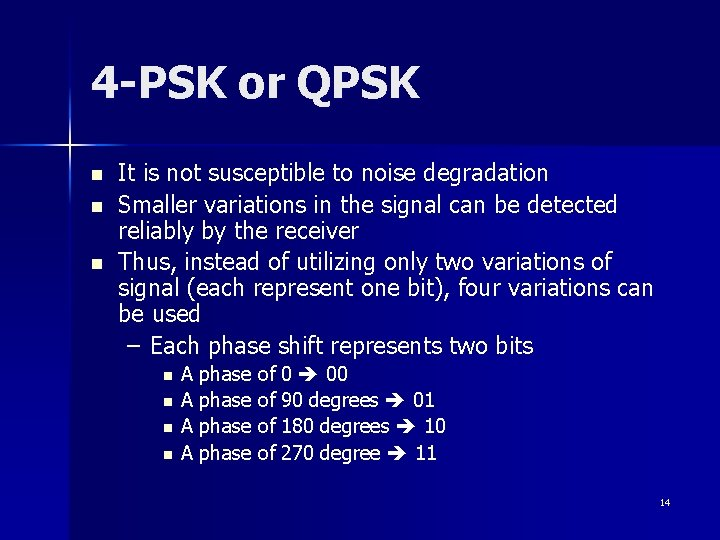4 -PSK or QPSK n n n It is not susceptible to noise degradation Smaller variations in the signal can be detected reliably by the receiver Thus, instead of utilizing only two variations of signal (each represent one bit), four variations can be used – Each phase shift represents two bits n n A phase of 0 00 A phase of 90 degrees 01 A phase of 180 degrees 10 A phase of 270 degree 11 14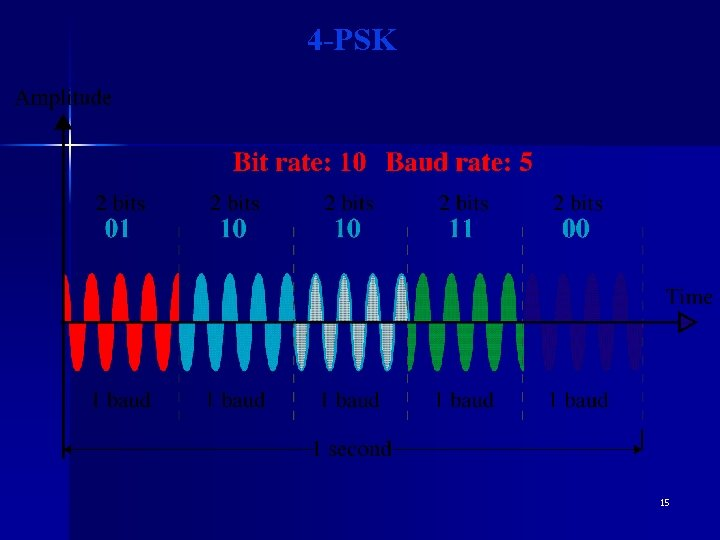4 -PSK 154 -PSK Characteristics 168 -PSK Characteristics The previous idea is extended to 8 -PSK 17PSK Bandwidth Minimum BW required is the same as ASK 18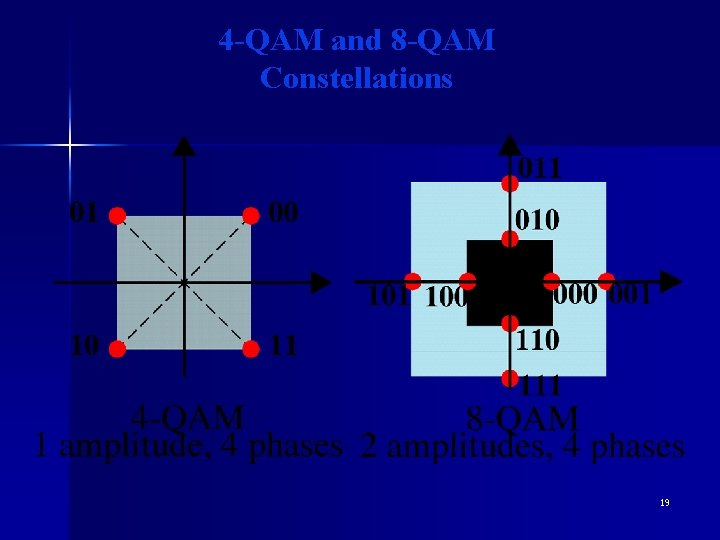4 -QAM and 8 -QAM Constellations 19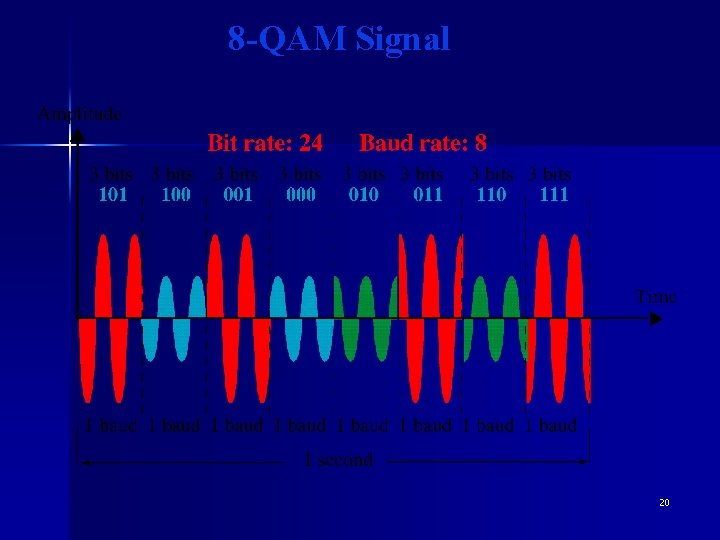8 -QAM Signal 20Aspects of Digital-to. Analog Conversion n Basic Issues – Bit rate – Baud rate – Carrier Signal 21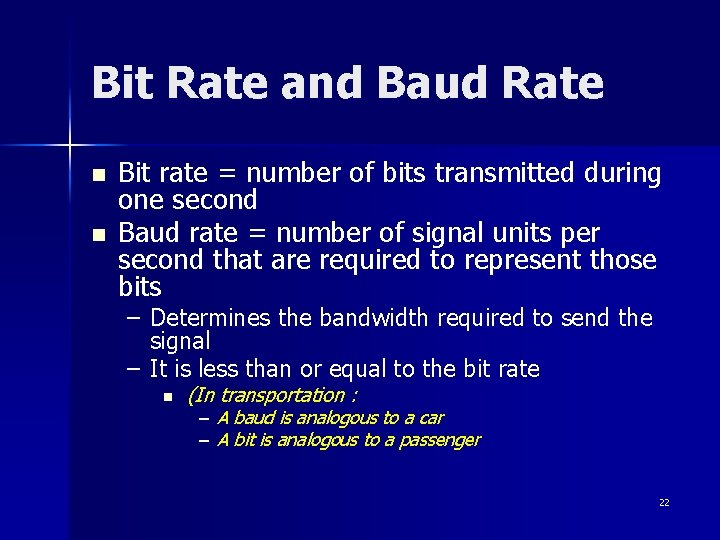Bit Rate and Baud Rate n n Bit rate = number of bits transmitted during one second Baud rate = number of signal units per second that are required to represent those bits – Determines the bandwidth required to send the signal – It is less than or equal to the bit rate n (In transportation : – A baud is analogous to a car – A bit is analogous to a passenger 22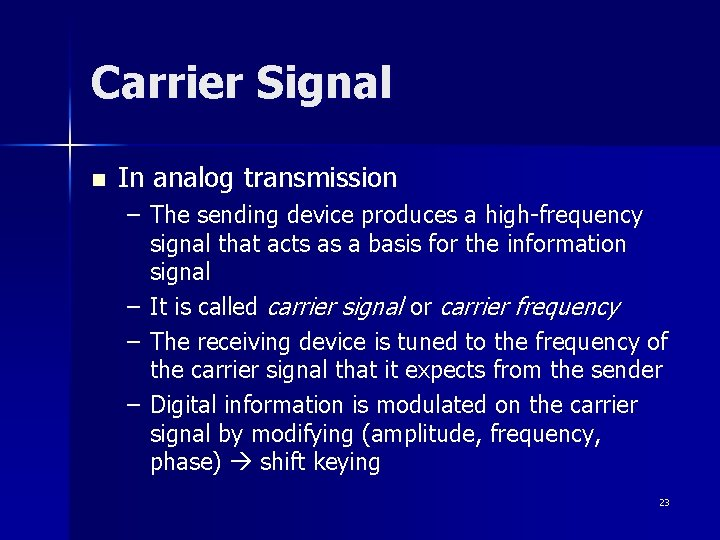Carrier Signal n In analog transmission – The sending device produces a high-frequency signal that acts as a basis for the information signal – It is called carrier signal or carrier frequency – The receiving device is tuned to the frequency of the carrier signal that it expects from the sender – Digital information is modulated on the carrier signal by modifying (amplitude, frequency, phase) shift keying 23Bit Rate and Baud Rate 24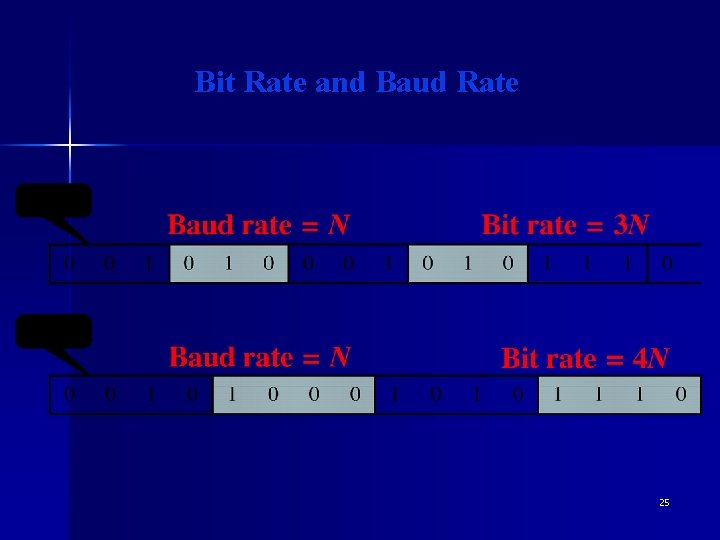Bit Rate and Baud Rate 25